Author: Oscar Cronquist Article last updated on February 11, 2018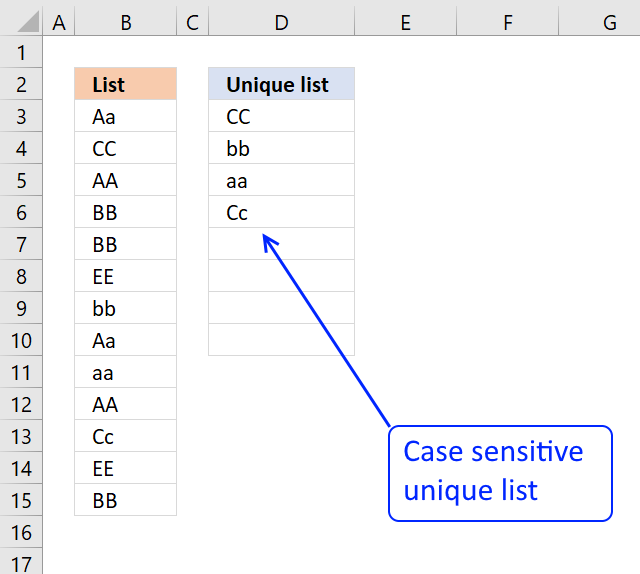My definition of unique values are values that exist only once in a cell range. The image below shows you a list in column B, some of these values have duplicates.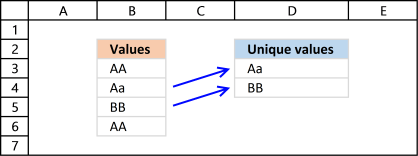The list in column D contains only values that are unique, Aa and BB exists only once in the list, all other values have a duplicate.

The formula extracts CC, bb, aa and Cc because they exist only once in column B.

Array formula in cell D3:

=INDEX(\$B\$3:\$B\$15, SMALL(IF(MMULT(EXACT(\$B\$3:\$B\$15, TRANSPOSE(B\$3:\$B\$15))*1, ROW(\$B\$3:\$B\$15)^0)=1, MATCH(ROW(\$B\$3:\$B\$15), ROW(\$B\$3:\$B\$15)), ""),ROWS(\$A\$1:A1)))

If you are looking for a unique distinct list, read this post: Extract unique distinct values (case sensitive) [Formula].

This post explains how to do a case-sensitive VLOOKUP and returning multiple values:

Case sensitive lookup and return multiple values

The array formula in cell F5 returns adjacent values from column C where values in column B matches the search […]

Make sure you read the following article if you want to extract duplicate values:

Extract a list of duplicates from a column

The array formula in cell C2 extracts duplicate values from column A. Only one duplicate of each value is displayed […]

### How to enter an array formula

1. Copy (Ctrl + c) above formula
2. Double click on cell C2
3. Paste (Ctrl + v) to cell C2
4. Press and hold CTRL + SHIFT simulatneously
5. Press Enter once
6. Release all keys

Your formula now looks like this: {=array_formula}
Don't enter the curly brackets, they appear automatically.

How to enter an array formula

Array formulas allows you to do advanced calculations not possible with regular formulas.

### Explaining array formula in cell D3:

You can easily follow along if you download the attached file and select cell D3. Then go to tab "Formulas" on the ribbon and click "Evaluate Formula" button.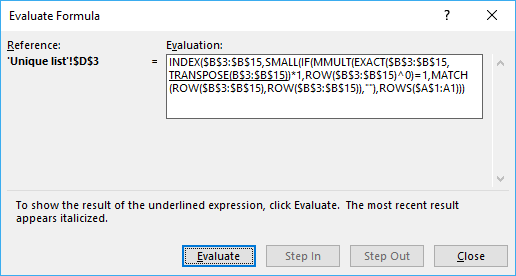Click "Evaluate" button shown in above picture to move to next step.

#### Step 1 - Check if values are case sensitive

The EXACT function is case sensitive function that allows you to compare values. If they match EXACT returns TRUE, if not FALSE.

EXACT(\$B\$3:\$B\$15,TRANSPOSE(B\$3:\$B\$15))*1

If we use TRANSPOSE we can compare values against each other to build an array, in a single calculation. The following picture shows you this array as an index table, I have highlighted cells that match green. Example, cell C3 shows you the result of a comparison between the value in cell C2 and B3. Since it is the same value they must match and the formula returns 1 and is highlighted green.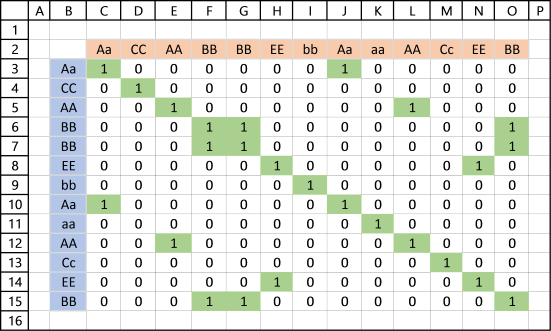It is now obvious that value Aa has a duplicate because cell J3 is also highlighted green.

Incredible that Excel allows you to do such a complicated calculation in a single cell.

How to use the TRANSPOSE function

The transpose function allows you to convert a vertical range to a horizontal range, or vice versa. A vertical range […]

#### Step 2 - MMULT function lets you SUM values column-wise or row-wise

The MMULT function is like the SUMPRODUCT function but on steroids, let me explain. SUMPRODUCT lets you multiply and then sum values, the result is a single value.

MMULT lets you multiply and sum values either column-wise or row-wise, the result is an array.

MMULT(EXACT(\$B\$3:\$B\$15, TRANSPOSE(B\$3:\$B\$15))*1, ROW(\$B\$3:\$B\$15)^0) is entered in column P, see picture below.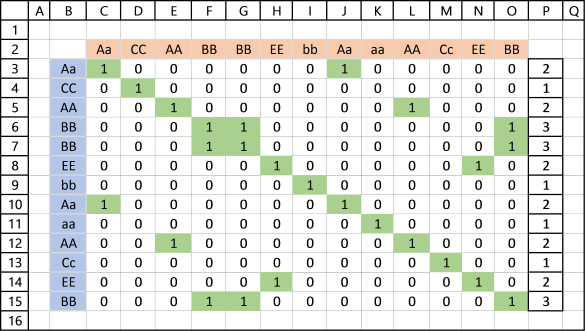It is now easy to spot unique values in the index table, if column P contains 1 the corresponding value in column B must be unique.

How to use the MMULT function

The MMULT function calculates the matrix product of two arrays, an array as the same number of rows as array1 and […]

#### Step 3 - Check if value in array is equal to 1 and if so return corresponding row number

Column Q shows you corresponding relative row number if value in column P is equal to 1.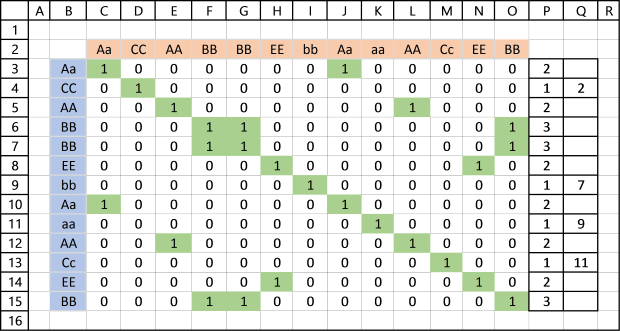IF(MMULT(EXACT(\$B\$3:\$B\$15, TRANSPOSE(B\$3:\$B\$15))*1, ROW(\$B\$3:\$B\$15)^0)=1, MATCH(ROW(\$B\$3:\$B\$15), ROW(\$B\$3:\$B\$15)), "")

returns this array: {"";2;"";""; "";"";7; "";9;"";11; "";""}

How to use the IF function

Checks if a logical expression is met. Returns a specific value if TRUE and another specific value if FALSE.

#### Step 4 - Filter the k-th smallest row number

SMALL(IF(MMULT(EXACT(\$B\$3:\$B\$15, TRANSPOSE(B\$3:\$B\$15))*1, ROW(\$B\$3:\$B\$15)^0)=1, MATCH(ROW(\$B\$3:\$B\$15), ROW(\$B\$3:\$B\$15)), ""),ROWS(\$A\$1:A1))

becomes

SMALL({"";2;"";""; "";"";7; "";9;"";11; "";""},ROWS(\$A\$1:A1))

becomes

SMALL({"";2;"";""; "";"";7; "";9;"";11; "";""},1)

and returns 2.

How to use the SMALL function

The SMALL function lets you extract a number in a cell range based on how small it is compared to the other numbers in the group.

#### Step 5 - Return value based on coordinate

INDEX(\$B\$3:\$B\$15, SMALL(IF(MMULT(EXACT(\$B\$3:\$B\$15, TRANSPOSE(B\$3:\$B\$15))*1, ROW(\$B\$3:\$B\$15)^0)=1, MATCH(ROW(\$B\$3:\$B\$15), ROW(\$B\$3:\$B\$15)), ""),ROWS(\$A\$1:A1)))

becomes

INDEX(\$B\$3:\$B\$15, 2)

becomes

INDEX({"Aa"; "CC"; "AA"; "BB"; "BB"; "EE"; "bb"; "Aa"; "aa"; "AA"; "Cc"; "EE"; "BB"}, 2)

and returns "CC" in cell D3.